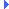FD.io VPP  v19.08-27-gf4dcae4 Vector Packet Processing
i2c.c File ReferenceInclude dependency graph for i2c.c:

Go to the source code of this file.

## Functions

static void i2c_delay (i2c_bus_t *b, f64 timeout)

static void i2c_wait_for_scl (i2c_bus_t *b)

static void i2c_start (i2c_bus_t *b)

static void i2c_stop (i2c_bus_t *b)

static void i2c_write_bit (i2c_bus_t *b, int sda)

static void i2c_read_bit (i2c_bus_t *b, int *sda)

static void i2c_write_byte (i2c_bus_t *b, u8 data)

static void i2c_read_byte (i2c_bus_t *b, u8 *data, int ack)

void vlib_i2c_init (i2c_bus_t *b)

void vlib_i2c_xfer (i2c_bus_t *bus, i2c_msg_t *msgs)

## Function Documentation

 static void i2c_delay ( i2c_bus_t * b, f64 timeout )
inlinestatic

Definition at line 20 of file i2c.c.Here is the call graph for this function:Here is the caller graph for this function:

 static void i2c_read_bit ( i2c_bus_t * b, int * sda )
static

Definition at line 90 of file i2c.c.Here is the call graph for this function:Here is the caller graph for this function:

 static void i2c_read_byte ( i2c_bus_t * b, u8 * data, int ack )
static

Definition at line 127 of file i2c.c.Here is the call graph for this function:Here is the caller graph for this function:

 static void i2c_start ( i2c_bus_t * b )
static

Definition at line 46 of file i2c.c.Here is the call graph for this function:Here is the caller graph for this function:

 static void i2c_stop ( i2c_bus_t * b )
static

Definition at line 63 of file i2c.c.Here is the call graph for this function:Here is the caller graph for this function:

 static void i2c_wait_for_scl ( i2c_bus_t * b )
static

Definition at line 27 of file i2c.c.Here is the call graph for this function:Here is the caller graph for this function:

 static void i2c_write_bit ( i2c_bus_t * b, int sda )
static

Definition at line 76 of file i2c.c.Here is the call graph for this function:Here is the caller graph for this function:

 static void i2c_write_byte ( i2c_bus_t * b, u8 data )
static

Definition at line 105 of file i2c.c.Here is the call graph for this function:Here is the caller graph for this function:

 void vlib_i2c_init ( i2c_bus_t * b )

Definition at line 150 of file i2c.c.Here is the caller graph for this function:

Definition at line 201 of file i2c.c.Here is the call graph for this function:Here is the caller graph for this function:

 void vlib_i2c_xfer ( i2c_bus_t * bus, i2c_msg_t * msgs )

Definition at line 168 of file i2c.c.Here is the call graph for this function:Here is the caller graph for this function: